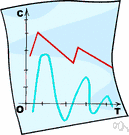# topological space

Also found in: Thesaurus, Acronyms, Encyclopedia, Wikipedia.
Related to topological space: metric space

## topological space

n.
A set of points together with a topology defined on them.
American Heritage® Dictionary of the English Language, Fifth Edition. Copyright © 2016 by Houghton Mifflin Harcourt Publishing Company. Published by Houghton Mifflin Harcourt Publishing Company. All rights reserved.

## topological space

n
(Mathematics) maths a set S with an associated family of subsets τ that is closed under set union and finite intersection. S and the empty set are members of τ
Collins English Dictionary – Complete and Unabridged, 12th Edition 2014 © HarperCollins Publishers 1991, 1994, 1998, 2000, 2003, 2006, 2007, 2009, 2011, 2014
ThesaurusAntonymsRelated WordsSynonymsLegend:
 Noun 1topological space - (mathematics) any set of points that satisfy a set of postulates of some kind; "assume that the topological space is finite dimensional"mathematical spaceinfinite, space - the unlimited expanse in which everything is located; "they tested his ability to locate objects in space"; "the boundless regions of the infinite"math, mathematics, maths - a science (or group of related sciences) dealing with the logic of quantity and shape and arrangementset - (mathematics) an abstract collection of numbers or symbols; "the set of prime numbers is infinite"subspace - a space that is contained within another spacenull space - a space that contains no points; and empty spacemanifold - a set of points such as those of a closed surface or an analogue in three or more dimensionsmetric space - a set of points such that for every pair of points there is a nonnegative real number called their distance that is symmetric and satisfies the triangle inequality
Based on WordNet 3.0, Farlex clipart collection. © 2003-2012 Princeton University, Farlex Inc.
Translations
topologický prostor
topologisk rum
topologinen avaruus
espace topologique
topološki prostor
spazio topologico
espaço topológico
References in periodicals archive ?
Both axioms M and G are valid in any particular point topological space.
A multilayer spatial model is a model that represents a specific space using several layers, such as a topological space layer and a sensor space layer, which are then combined .
When S is semi-topological, let S : S x X [right arrow] X be a representation of S on a topological space X.
The pair (f, [??]) is called a soft topological space over f and the members of [??] are said to be soft open in f.
A topologized group is a triple (Eq.) such that (Eq.) is a group and (Eq.) is a topological space. If both the multiplication (Eq.) and the inversion (Eq.) appings of (Eq.) are continuous, then (Eq.) is called a topological group.
Throughout this paper (X,[tau]) and (X,[[tau].sub.[alpha]]) will always be topological spaces. For a subset A of topological space X, Int(A), Cl(A), [Cl.sub.[alpha]](A) and [Int.sub.[alpha]](A) denote the interior, closure, [alpha]-closure and [alpha]-interior of A respectively and [G.sub.[alpha]] is the [alpha]-open set for topology [[tau].sub.[alpha]] on X.
In the words of Belcher and his colleagues, we have been offered, theoretically as well as empirically, the chance to consider the camp as a topological space, that is to say, as a spatiality that is "always potential--that is, both capable of becoming and not becoming" (2008, page 502).
The subject of ideals in topological space has been studied by Kuratowski  and Vaidyanathaswamy .
The pair (L, [tau]) is called a topological space. A subset B of [tau] is called a base of [tau], if for each A [member of] [tau] and each x [member of] A, there exists B [member of] B such that x [member of] B [subset or equal to] A.
Let (X, [mu]) be a generalized topological space. Then
These entities could be considered points of a directed axis, he says--in temporal cases they could be time points, time intervals, or more complex entities; and in spatial cases could be points of the plane, regions of the plane, pairs of points, or even subspaces of a topological space. His topics include polynomial subclasses of Allen's algebra, binary qualitative formalisms, fuzzy reasoning, a categorical approach of qualitative reasoning, and applications and software tools.
A topological space (X, [tau]) is said to be sg*-normal if for any pair of disjoint sg-closed subsets F1 and F2 of X, there exist disjoint open sets U and V such that F1 [subset] U and F2 [subset] V .

Site: Follow: Share:
Open / Close